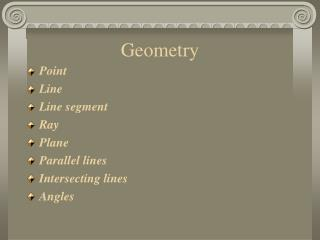DownloadDownload PresentationGeometry

# Geometry

Download Presentation## Geometry

- - - - - - - - - - - - - - - - - - - - - - - - - - - E N D - - - - - - - - - - - - - - - - - - - - - - - - - - -
##### Presentation Transcript

1. Geometry • Point • Line • Line segment • Ray • Plane • Parallel lines • Intersecting lines • Angles

2. Point An exact location in space Line An endless collection of points along a straight path Line segment Part of a line that has two endpoints .A Geometry

3. Ray Part of a line that has one endpoint and extends endlessly in the other direction Plane An endless, flat surface that is named by any three points not on the same line. Geometry .A .C .B

4. Parallel Lines Lines do not intersect but are in the same plane Intersecting Lines Lines meet at one point Perpendicular Lines Lines form a right angle Geometry

5. Angles • An angleis formed when two rays have the same endpoint. • This endpoint is called the vertex. • The two rays that form the angle are called sides.

6. Angles • There are four types of angles • Right angle • Straight angle • Acute angle • Obtuse angle

7. Forms a square corner Forms a 90 degree angle. Use a corner of your index card to make sure you a have a right angle Right Angles 90 degrees

8. Forms a straight line Angle is 180 degrees Use the edge of your index card to make sure you have a straight angle. 180 degrees Straight Angle

9. Forms an angle that is less than a right angle Angle is less than 90 degrees Acute Angles

10. Form an angle that is more than a right angle Angle is more than 90 degrees Obtuse Angles

11. Ray Parallel lines Line 1. ReviewName each picture 2. 3.

12. Acute angle Line segment Point ReviewName each picture 4. 5. 6.

13. ReviewName each picture Intersecting lines Perpendicular and Intersecting lines Plane